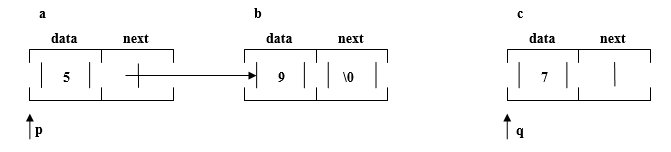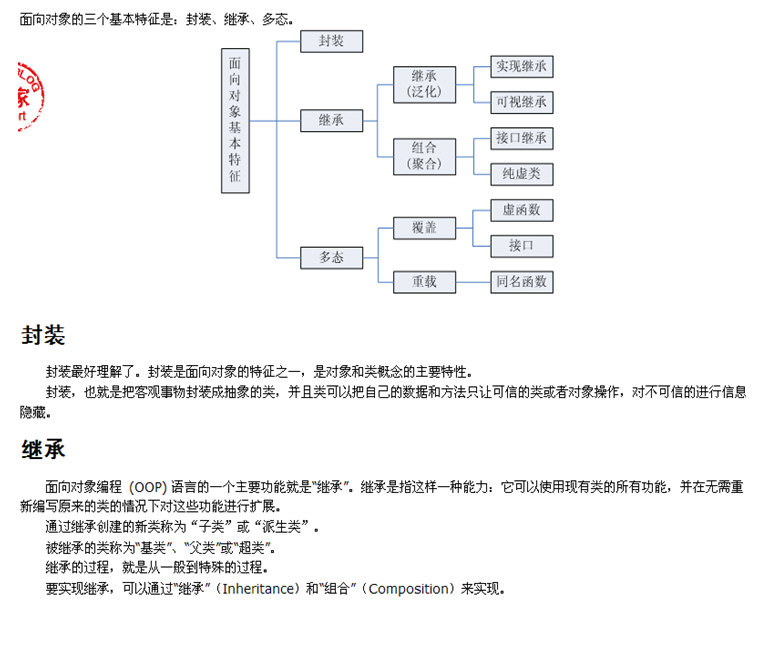## 华中科技大学软件工程复试考研真题大全(2007-2010)

###### 点赞(0) 反对(0) 本站小编 福瑞考研网 2017-04-04 21:33:10 阅读(0)

：以下代码或是我自己写的，或是经过修改并验证是正确的，全部用C语言编写，仅供参考。813题不明来路，9题以后主要是数据结构的题，没有提供答案，个人认为不会出这么难，更主要原因是，我也不会做……O(_)O~红色部分为注释重点代码

201020071、任意输入一串字符，将下标为奇数的小写字母转换为大写（编号从0开始，若该位置上不是字母，则不转换）。举例：若输入abc4Efg,则应输出aBc4EFg(字符串数组)

# include<stdio.h>

# include<string.h>

# define MAX 20

void main()

{

void fun(char *s);

char ss[MAX];

gets(ss);

fun(ss);

printf("\n%s\n",ss);

}

void fun(char *s)

{

int i,k=strlen(s);

for(i=1;i<k;i=i+2)

if(s[i]>='a' && s[i]<='z')

s[i]-=32;

}

20102、在半个中国象棋棋盘上,马在左下角(11),马走日字...而且只能往右走...不能向左...可上可下...求从起点到(m, n)处有几种不同的走法（函数的递归调用）

#include <stdio.h>

int horse(int x1,int y1,int x2,int y2)

{

int result=0;

if (y1>y2 || y1==y2 && x1!=x2)

return 0;

else if (x1==x2 && y1==y2)

return 1;

else

{

if (x1-1>0 && y1+2<=9)

result+=horse(x1-1,y1+2,x2,y2);

if (x1-2>0 && y1+1<=9)

result+=horse(x1-2,y1+1,x2,y2);

if (x1+2<=5 && y1+1<=9)

result+=horse(x1+2,y1+1,x2,y2);

if (x1+1<=5 && y1+2<=9)

result+=horse(x1+1,y1+2,x2,y2);

return result;

}

}

void main()

{

int m,n;

printf("请输入目的地址：\n");

scanf("%d%d",&m,&n);

while (m>5 || m<1 || n>9 || n<1) //半个中国象棋的边界

{

printf("输入错误，请重新输入：");

scanf("%d%d",&m,&n);

}

printf("目的结果数为%d\n",horse(1,1,m,n));

}

20093、给定任意俩组字符串S1S2，请编程输出他们间的最大相同子串。例如：S1=12abc78

S2=7bc2，则输出为：bc (字符串数组)

# include <stdio.h>

# include <string.h>

# define MAX 20

void main()

{

void search_s(char *s1,char *s2);

char s1[MAX],s2[MAX];

printf("please input string 1:\n");

gets(s1);

printf("please input string 2:\n");

gets(s2);

search_s(s1,s2);

}

void search_s(char *s1,char *s2)   //记录相同字符串

{

int print_s(int *s1,int *s2,int p);

int i,j,s=strlen(s1),t=strlen(s2);

int s3[MAX]={0},s4[MAX],p=0,k;

int d;

for(i=0;i<s;i++)

{

for(j=0;j<t;j++)

if(s1[i]==s2[j])

{

s4[p]=i; //记录相同字符串起始字符位置

s3[p]=1;p++;//记录连续相同字符个数

for(k=1;s1[i+k] && s2[j+k];k++)

{

if(s1[i+k]==s2[j+k])

s3[p-1]=s3[p-1]+1;

}

}

}

d=print_s(s3,s4,p);

for(i=s4[d];i<s4[d]+s3[d];i++)

printf("%c",s1[i]);

printf("\n");

}

int print_s(int *s1,int *s2,int p)     //找到打印位置

{

int i,max=s1,k;

for(i=1;i<p;i++)

{

if(max<s1[i])

{

max=s1[i];

k=i;

}

}

return k;

}

200920084、已知一颗二叉树S的前序遍历和中序遍历序列，请编程输出二叉树S的后续遍历序列。

(举例：pred[]/先序：ABDECFG

inod[]/中序：DBEACGF

#include <stdio.h>

#include<string.h>

int find(char c,char A[],int s,int e) //找出中序中根的位置

{

int i;

for(i=s;i<=e;i++)

if(A[i]==c) return i;

}

void pronum(char pre[],int pre_s,int pre_e,char in[],int in_s,int in_e)

{

char c;

int k;

if(in_s>in_e) return ; //非法子树，完成

if(in_s==in_e)

{

/* 子树子仅为一个节点时直接输出并完成*/

printf("%c ",in[in_s]);

return ;

}

c=pre[pre_s];           // c储存根节点

/* 在中序中找出根节点的位置*/

k=find(c,in,in_s,in_e);

/* 递归求解分割的左子树，请特别关注参数的确定！*/

pronum(pre,pre_s+1,pre_s+k-in_s,in,in_s,k-1);

/* 递归求解分割的右子树，请特别关注参数的确定！*/

pronum(pre,pre_s+k-in_s+1,pre_e,in,k+1,in_e);

printf("%c ",c);  //根节点输出

}

void main()

{

char pre[]="ABDEGCZFHI";

Charin[]="DBGEAZCHFI";

printf("The result:");

pronum(pre,0,strlen(in)-1,in,0,strlen(pre)-1);

printf("\n");

}

20085、打印出所有的水仙花数，所谓水仙花数是指一个3位数，其各位数字立方和等于该数本身。

(举例：153=1*1*1+3*3*3+5*5*5)（多层循环）

#include<stdio.h>

void main()

{

int i,j,k;

for(i=1;i<=9;i++)

for(j=0;j<=9;j++)

for(k=0;k<=9;k++)

{

if((i*100+j*10+k)==(i*i*i+j*j*j+k*k*k))

{

printf("%5d",i*100+j*10+k);

}

printf("\n");

}

}

2008,20076、求数列s(n)=s(n-1)+s(n-2)的第n项的值。其中s(1)=s(2)=1。要求任意给定n,输出s(n)(递归基础)

#include<stdio.h>

void main()

{

int s(int n);

int k;

printf("input k:");

scanf("%d",&k);

printf("s(%d)=%d\n",k,s(k));

}

int s(int n)

{

if(n==1 || n==2)

return 1;

else

return s(n-1)+s(n-2);

}

20077、按要求输出：在三位整数（100999）中寻找符合条件的整数并依次从小到大存入数组中；他既是完全平方数，又是两位数字相同，例如144676（多位数字的分离，注意与第5题比较）

#include<stdio.h>

#define MAX 20

void main()

{

int i,j,k=0;

int a[MAX],*p;

p=a;

for(i=100;i<1000;i++)

for(j=0;j<100;j++)

{

int a,b,c;

a=i%10;

b=i/10%10;

c=i/100;

if((a==b||a==c||b==c)&&(i==j*j))

{

*p++=i;

k++;

}

}

for(i=0;i<k;i++)

printf("%d\n",a[i]);

}

8、在一个整形数组a中既有负数又有正数，编写一个算法a中所有负数移到整数之前，要求其时间复杂度为O(n)n为数组长度，并且只使用常数个辅助空间。（辅助空间的使用，数组元素的选择性保留）

#include<stdio.h>

void main()

{

int i,j=0,temp;

int a={1,2,3,4,-1,1,-2,-1,-4};

for(i=8;i>=0;i--)

{

if(a[i]<0)

{

temp[j]=a[i];//拿出负数元素

j=j+1;

a[i]=0;//在原数组中抛弃负数元素

}

}

for(i=0;i<9;i++)

if(a[i]!=0)

temp[j++]=a[i];//取出正数元素

for(i=0;i<9;i++)

{

a[i]=temp[i]; //完成后元素回归，注意读题

printf("%d ",a[i]);

}

printf("\n");

}

9、编写一个C函数，输入一个二叉树的根节点，返回这棵树中所有值大于0的节点值之和，如果根为空，返回0.

Typedef struct node{

ElemType data;

struct node *lchild;

struct node *rchild;

}BTree;

10A是一个长度为N的整形数组，其中可能包含重复的元素，例如A={1,2,2,3,2,1,3,2}，删除数组中相同的元素后得到{1,2,3}

a) 如果数组没有排序，写一个C语言函数，输入参数为数组首地址和长度，删除其中重复的元素，返回删除后的数组的长度。

b) 上述函数的时间复杂度为多少，以删除前的数组长度N表示。

c) 如果数组A已经排好序，设计并写出一个C语言函数完成a)中的工作，要求时间复杂度是O(N)

11、写一个C语言函数将一颗二叉树用层序遍历列出所有节点，即先列出根节点，再从左向右列出深度为1的节点的值，然后再左向右列出深度为2的节点的值，如此继续。数的节点类型TREENODE包含一个整型值Value和俩个指针：LeftChildRightChild。可以使用的函数（不限于）包括MaleEmptyQueue (QUEUE *q),

EnQueue (QUEUE *q,TREENODE *tn)

DeQueue (QUEUE *q,TREENODE *tn) , IsEmpty (QUEUE *q) , DisposeQueue (QUEUE *q)

12、假设以下关于堆栈的库函数已经给出，unsigned char is empty ();检查堆栈是否为空，如果是，返回1；否则返回0. void push (char element);把一个char型的数据element 推入栈顶。

Char pop (); 弹出栈顶的char型数据。

（1）  利用这些库函数设计一个C语言的函数unsigned char isBalanced (char *string) ,来检查字符串string 中的括号（），[]{}是否平衡，如果平衡，该函数返回1，否则返回0.

（2）  你所设计的函数时间复杂性是多少（假定字符串string 长度为n）？

13、在一棵高度为O(logn)的二叉排序树的结点上存储着浮点数，请用C语言写一个函数来计算一棵树上界于任意俩个浮点数x1x2 (x1<x2)之间的结点数。说明你的算法的计算复杂度，算法计算复杂度越低越好。

2008说明：红色部分为注释，蓝色部分为答案，原题没有；大红色部分为原题中需要注意的地方

1  D  是合法的用户自定义标识符。

A. b-b               B. float                C. <fr>                      D. _isw

2  C  xy定义成float类型变量，并赋同一初值3.14

A. float x,y=3.14    B. float x,y=2*3.14     C. float x=3.14,y=x=3.14     D. float x=y=3.14

3、表达式sizeof（“hello”）strlen（“hello”）的值分别是  C  sizeof求实际存储空间strlen求字符个数，不包括结束符）

A.6,6                B. 5,5                  C. 6,5                       D. 5,6

4、以下程序输出的结果是  D  （静态局部变量）

int f()

{

static int i=0;

static int s=1;

s+=i;i++;

return s;

}

main()

{

int i,a=0;

for(i=0;i<5;i++)

a+=f();

printf(“%d\n”,a);

}

A. 10                B. 15                    C. 20                        D. 25

5、已知一棵二叉树的前序遍历和中序遍历分别为ABDEGCFHDBGEACHF，则该二叉树的后序遍历为  B

A. GEDHFBCA          B. DGEBHFCA              C. ABCDEFGH                 D. ACBFEDHG

6、以下程序的输出结果是  A  case语句的执行特点）

main()

{

int a=0,i;

for(i=1;i<5;i++)

{

swich(i)

{

case 0:

case 3:a+=2;

case 1:

case 2:a+=3;

default:a+=5;

}

}

printf(“%d\n”,a);

}

A. 31                 B. 13                     C. 10                      D. 20

7、设有int a[]={10,11,12}*p=&a;则执行完*p++; *p+=1;后，a, a, a的值依次是  C  (符号运算的优先级，*p++=*p++），即指针右移一位)

A. 10,11,12           B. 11,12,12               C. 10,12,12                D. 11,11,12

8、以下程序的输出结果是  B  （二维数组的初始化）

main()

{

char ch={“AAAA”,”BBB”,”CC”};

printf(“\”%s\”\n”,ch);//特殊字符的输出，但此题并没有考这一点，选项均含双引号

}

A. “AAAA”            B. “BBB”                C. “BBBCC”               D. “CC”

9、在C语言中，形参的缺省存储类是  A

A. auto                B. register               C. static                  D. extern

10、若以下定义：

struct link

{

int data

struct link *next

}abc*p*qA. a.next=c; c.next=b;                 B. p.next=q; q.next=p.next;

C. q->next=p->next; p->next=&c;        D. (*p).next=q; (*q).next=&b;

1、以下程序的输出结果是  s=8  （注意输出的形式）

main（）

{

int a={{3,2,1},{4,5,6},{2,9,2}};

int i,j,s=0;

for(i=0;i<3;i++)

for(j=0;j<3;j++)

if(i==2-j) s=s+a[i][j];

printf(”s=%d”,s);

}

2#include<stdlib.h>

struct NODE{

int num;

struct NODE *next;

};

main()

{

struct NODE *p,*q,*r;

int sum=0;

/* 请求分配内存空间*/

p=(struct NODE *)malloc(sizeof(struct NODE));

q=(struct NODE *)malloc(sizeof(struct NODE));

r=(struct NODE *)malloc(sizeof(struct NODE));

/* 赋值，建立链表*/

p->num=1; q->num=2; r->num=3;

p->next=q; q->next=r; r->next=NULL;

sum+=q->next->num; sum+=p-> num;

printf(“%d\n”,sum);

}执行后的输出结果是  4

3、阅读以下C程序，将程序的全部输出逐行写在答卷上。

[程序] int test4(int n)

{

int d=0,m=n;

while(m)

{

d=d*10+m%10;

m/=10;

}

return d= =n;

}

int data[]={5,123,121,453,545};

main()

{

int I;

for(I=0;I<sizeof data/sizeof(int);I++)

printf(“%5d:%s\n”,data[I],test4(data[I]?”OK”:”NO”));

}

5:OK

123:NO

121:OK

453:NO

 定  义 含              义 int i； 定义整型变量i int *p； 0p为指向整型变量的指针变量 int a[n]； 定义整型数组a，它有n个元素 int *p[n]； 定义指针数组p，它由n个指向整型数据的指针元素组成 int (*p)[n]； p为指向含有n个元素的一元数组的指针变量 int f()； f为返回整型数值的函数 int *p()； p为返回一个指针的函数，该指针指向整型数据 int (*p)()； p为指向函数的指针，该函数返回一个整型值 int **p； p是一个指针变量，它指向一个指向整型数据的指针变量

20091、谈一下你做软件项目的经历。采用了哪些工具和方法？（自己发挥，这是我的经历，仅供参考，O(_)O~

Myeclipse + SQL server 2005 + CVS

Tomcat

Project 2003

Visio 2003

2、简要介绍你所熟悉的一种编程工具或开发环境（自己发挥，这是我的经历，仅供参考，O(_)O~

MyEclipse，是一个十分优秀的用于开发Java, J2EEEclipse插件集合，MyEclipse的功能非常强大，支持也十分广泛，尤其是对各种开元产品的支持十分不错。

MyEclipse企业级工作平台（MyEclipse Enterprise Workbench ，简称MyEclipse）是对Eclipse IDE的扩展，利用它我们可以在数据库和JavaEE的开发、发布，以及应用程序服务器的整合方面极大的提高工作效率。它是功能丰富的JavaEE集成开发环境，包括了完备的编码、调试、测试和发布功能，完整支持HTML, Struts, JSF, CSS, Javascript, SQL, Hibernate

1. JavaEE模型

2. WEB开发工具

3. EJB开发工具

4. 应用程序服务器的连接器

5. JavaEE项目部署服务

6. 数据库服务

7. MyEclipse整合帮助

对于以上每一种功能上的类别，在Eclipse中都有相应的功能部件，并通过一系列的插件来实现它们。MyEclipse结构上的这种模块化，可以让我们在不影响其他模块的情况下，对任一模块进行单独的扩展和升级。

简单而言，MyEclipseEclipse的插件，也是一款功能强大的JavaEE集成开发环境，支持代码编写、配置、测试以及除错，MyEclipse6.0以前版本需先安装EclipseMyEclipse6.0以后版本安装时不需安装Eclipse

20093、面向对象的三大特征和意义是什么。